#### STAT 801

Problems: Assignment 2

Suppose X and Y have joint density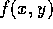. Prove from the definition of density that the density of X is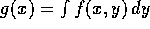.

Suppose X is Poisson(). After observing X a coin landing Heads with probability p is tossed X times. Let Y be the number of Heads and Z be the number of Tails. Find the joint and marginal distributions of Y and Z.

Let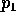be the bivariate normal density with mean 0, unit variances and correlation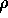and let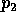be the standard bivariate normal density. Let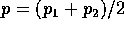.

1. Show that p has normal margins but is not bivariate normal.

2. Generalize the construction to show that there rv's X and Y such that X and Y are each standard normal, X and Y are uncorrelated but X and Y are not independent.

Warning: This is probably hard. Don't waste too much time on it. Suppose X and Y are independent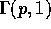and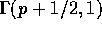random variables. Show that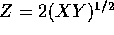is a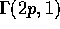random variable.

Suppose X and Y are independent with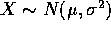and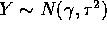. Let Z=X+Y. Find the distribution of Z given X and that of X given Z.

Richard Lockhart
Thu Oct 10 22:10:13 PDT 1996